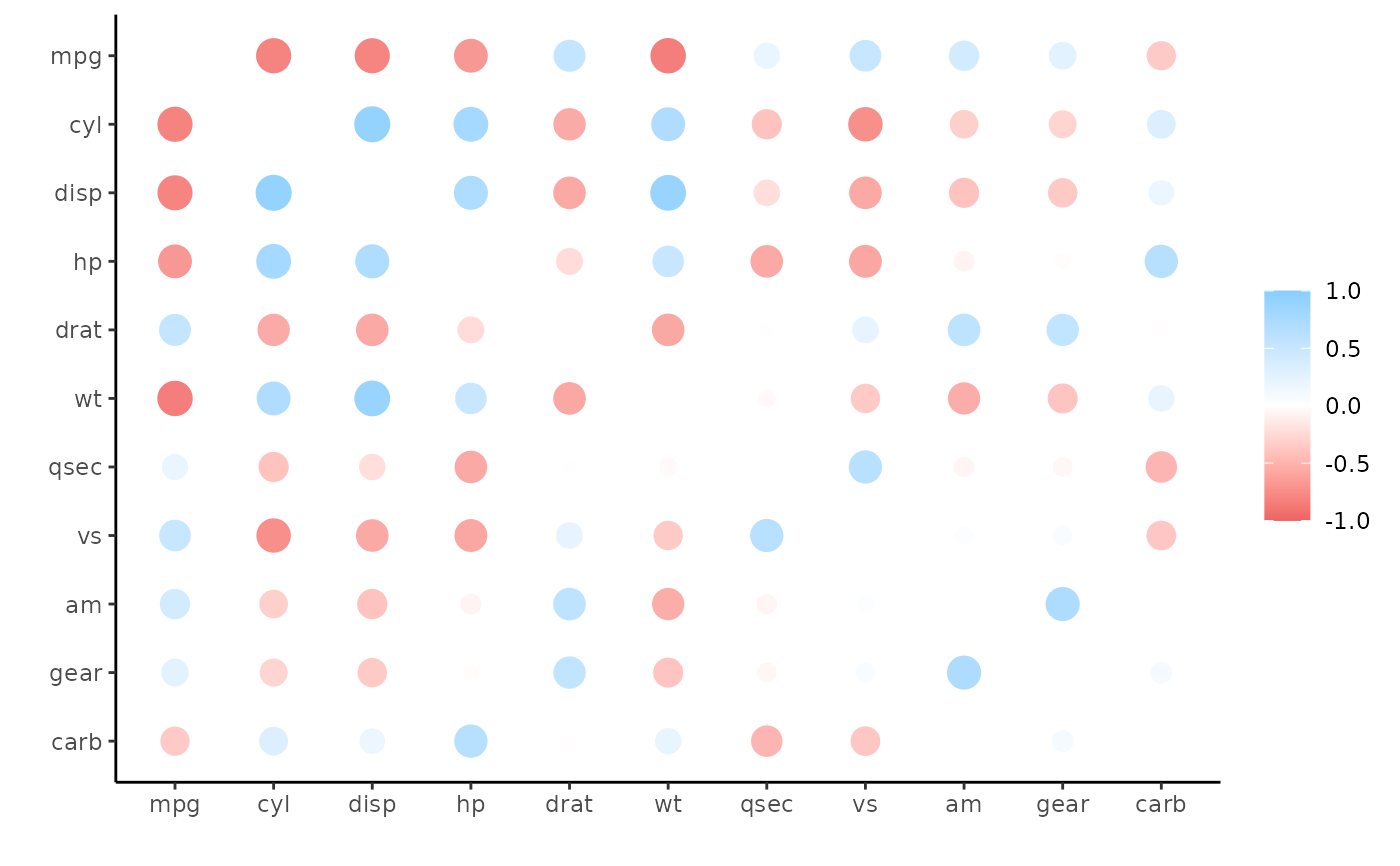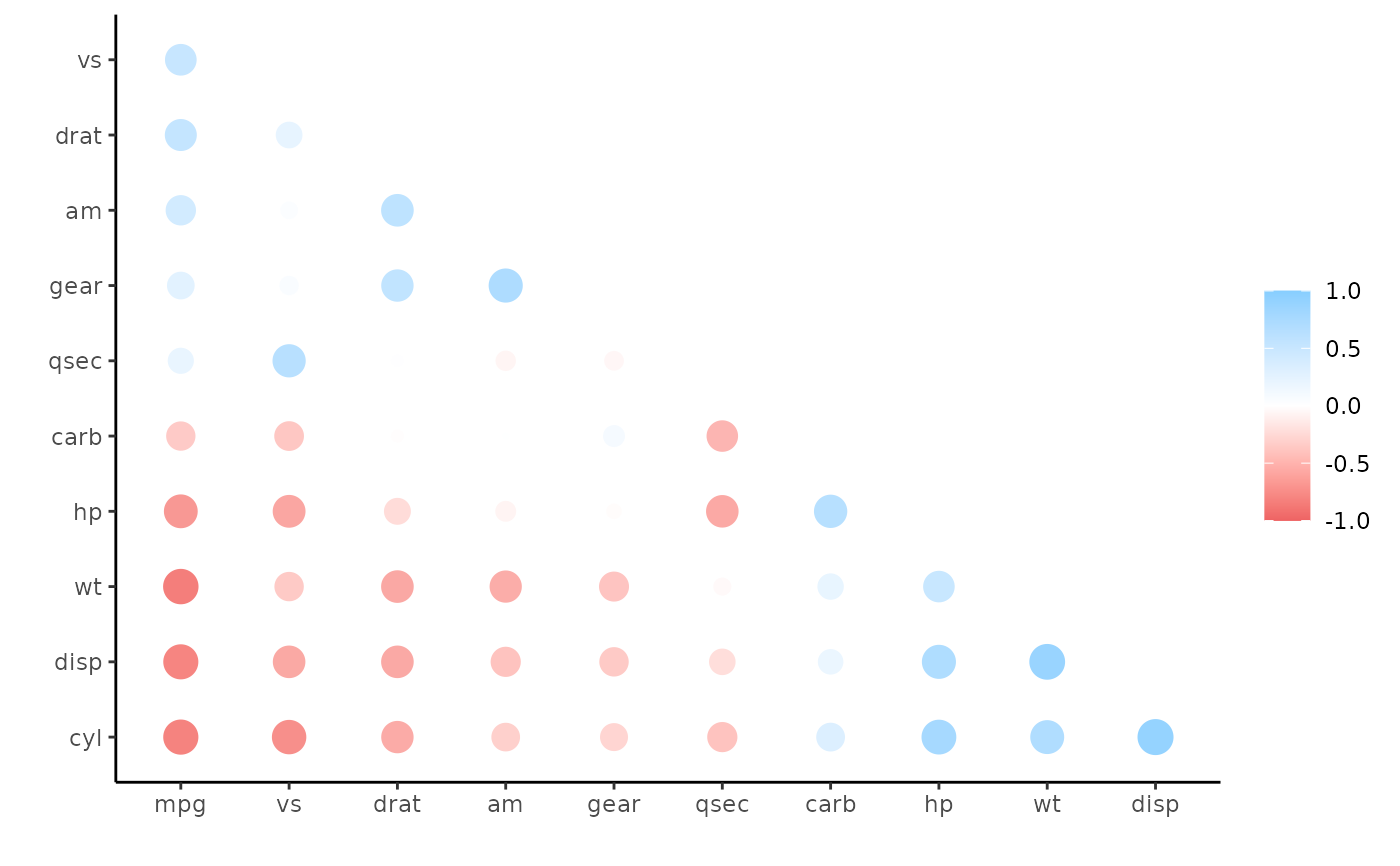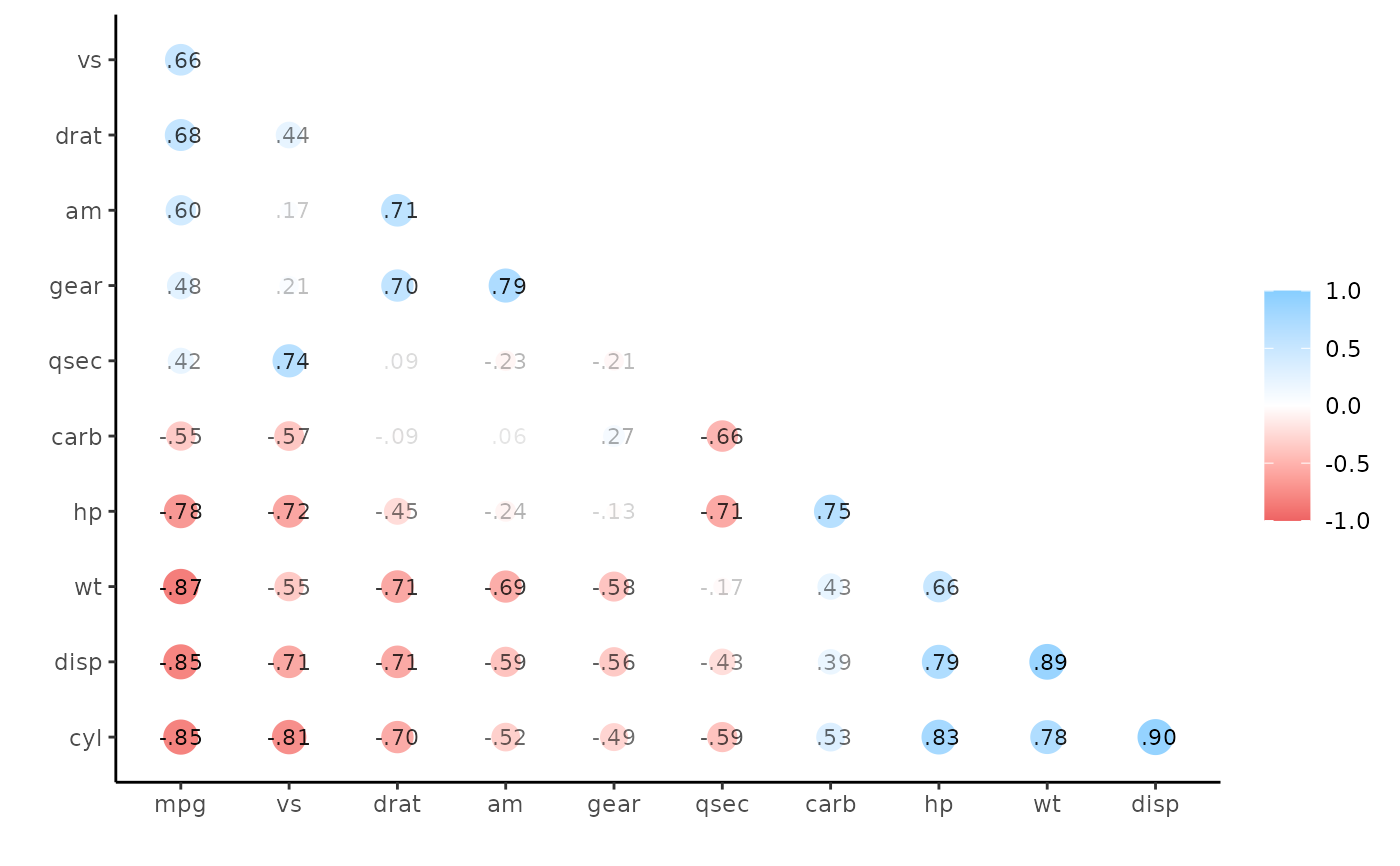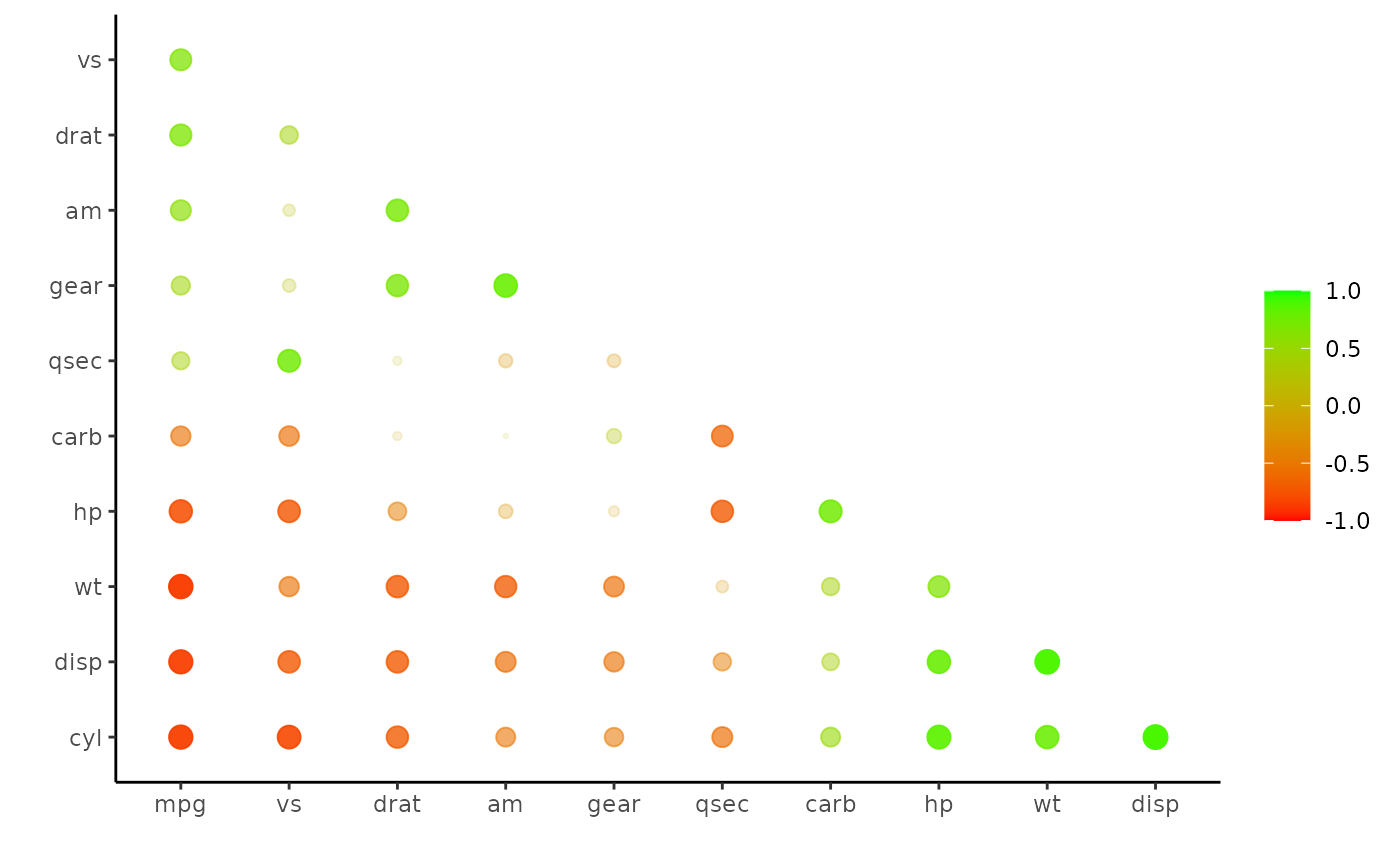Plot a correlation data frame using ggplot2.

## Usage

rplot(
rdf,
legend = TRUE,
shape = 16,
colours = c("indianred2", "white", "skyblue1"),
print_cor = FALSE,
colors,
.order = c("default", "alphabet")
)

## Arguments

rdf

Correlation data frame (see correlate) or object that can be coerced to one (see as_cordf).

legend

Boolean indicating whether a legend mapping the colors to the correlations should be displayed.

shape

geom_point aesthetic.

colours, colors

Vector of colors to use for n-color gradient.

print_cor

Boolean indicating whether the correlations should be printed over the shapes.

.order

Either "default", meaning x and y variables keep the same order as the columns in x, or "alphabet", meaning the variables are alphabetized.

## Value

Plots a correlation data frame

## Details

Each value in the correlation data frame is represented by one point/circle in the output plot. The size of each point corresponds to the absolute value of the correlation (via the size aesthetic). The color of each point corresponds to the signed value of the correlation (via the color aesthetic).

## Examples

x <- correlate(mtcars)
#> Correlation computed with
#> • Method: 'pearson'
#> • Missing treated using: 'pairwise.complete.obs'
rplot(x)# Common use is following rearrange and shave
x <- rearrange(x, absolute = FALSE)
x <- shave(x)
rplot(x)rplot(x, print_cor = TRUE)rplot(x, shape = 20, colors = c("red", "green"), legend = TRUE)